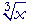An Approach

to

C A L C U L U S

6

# DERIVATIVES

The derivative of a constant

THE DEFINTION of the derivative is fundamental. (Definition 5.) The student should be thoroughly familiar with it. From that definition it is possible to prove various rules, some of which we will present in this Lesson. The student will find it extremely helpful to state each rule verbally.

For example,

 1"The derivative of a constant is 0."

We should expect this because the slope of a horizontal line  y = c  is 0.

 2"The derivative of a variable with respect to itself is 1."

Again, this is an expected result, because 1 is the slope of the straight line y = x.  (Topic 9 of Precalculus.)

 3"The derivative of a sum or difference
is equal to the sum or difference of the derivatives."

This follows from Theorem 1 on limits, Lesson 2.

For example,

 d dx (x2 − x + 5)  =  2x − 1,

according to the Theorem of Lesson 4, and 1, 2, and 3 above.

 4 d dx c f(x)  =  c d dx f(x)

"The derivative of a constant times a function
is equal to the constant times the derivative of the function."

 d dx 5x2  =  5· 2x  =  10x. d dx 8x  =  8· 1 =  8.

This follows from Theorem 5 on limits, Lesson 2.

Problem 1.   Calculate the derivative of  4x2 − 6x + 2.

Do the problem yourself first!

8x − 6.

 Problem 2.   Calculate  the derivative of  y = x a , where a is a constant.
 Since xa = 1a x (Lesson 5 of Algebra), then y' = 1a .

Rule 4.

 5 ( f g)'  =  f g' + g f '

"The derivative of a product of two functions is equal to

the first times the derivative of the second
plus the second times the derivative of the first."

This is the product rule.  We will prove it below.

Example.   Accepting for the moment that the derivative of  sin x is cos x (Lesson 12), then

 d dx x2 sin x  =  x2 cos x  +  2x sin x.

Problem 3.   Calculate the derivative of  5x sin x.

5x cos x + 5 sin x

The power rule

 6 d dx xn  =  nxn−1

"The derivative of a power of x

is equal to the product of
the exponent  times
x with the exponent reduced by 1."

That is called the power rule.  For example,

 d dx x5 =  5x4

It is usual to prove the power rule by means of the binomial theorem. See Topic 24 of Precalculus, especially Problem 5.  On applying the definition of the derivative, subtracting xn, dividing the numerator by h and taking the limit, the rule follows.

However, we have seen that the power rule is true when n = 1:

 d dx x1 =  1· x0  =  1; it is true when n = 2: d dx x2 =  2x; and that it is true when n = 3 (Problem 2 of Lesson 5): d dx x3 =  3x2.

It seems natural, then, to give a proof by induction; (Topic 26 of Precalculus).  The induction hypothesis will be that the power rule is true for n = k:

 d dx xk =  k xk−1,

and we must show that it is true for n = k + 1; i.e. that

 d dx xk+1 =  (k + 1) xk.

Now,

 d dx xk+1 = d dx x· xk
 d dx xk+1 = x· k xk−1 + xk· 1,

according to the product rule and the induction hypothesis;

 d dx xk+1 = k xk + xk, Rule 1 of exponents, d dx xk+1 = (k + 1)xk.

Therefore, if the power rule is true for n = k, then it is also true for its successor, k + 1.  And since the rule is true for n = 1, it is therefore true for every natural number.

Problem 4.   Calculate the derivative of  x6 − 3x4 + 5x3x + 4.

6x5 − 12x3 + 15x2 − 1

We will see in Lesson 14 that the power rule is valid for any rational exponent n.  The student should begin immediately to use that result.

Example.  The derivative of the square root.See Lesson 29 of Algebra:  Rational Exponents.

Problem 5.   Calculate the derivative of.Problem 6.   Calculate the derivative of x.Problem 7.   Calculate the derivative of 1 x5 .
 d dx 1 x5 = d dx x−5 = −5x−6

Proof of the product rule

To prove the product rule, we will express the difference quotient simply

 as ΔyΔx .  (Lesson 5.)  And so let

y = f g.

Then a change in y -- Δy -- will produce corresponding changes in  f and g:

y + Δy = (f  + Δf )(g + Δg)

On multiplying out the right-hand side,

y + Δy = f g  +  f Δg  +  g Δf  +  Δf Δg.

 To form ΔyΔx , we subtract y -- which is f g -- from both sides, and

divide by Δx:

 ΔyΔx =  f ΔgΔx +  g Δf Δx +  Δf ΔgΔx

Now let Δx0.  Hence Δy  will approach 0, as will both Δf and Δg, so that the last term on the right approaches 0.

Therefore, since the limit of a sum is equal to the sum of the limits (Theorem 1 of limits):

 dydx = f dgdx + g dfdx

This is the product rule.Next Lesson:  The chain rule

Please make a donation to keep TheMathPage online.
Even \$1 will help.